Mathematics Commons™

16,960 Full-Text Articles 16,272 Authors 4,137,002 Downloads277 Institutions

All Articles in Mathematics

16,960 full-text articles. Page 482 of 491.

Mathematical Reasoning In Service Courses: Why Students Need Mathematical Modeling Problems, 2010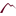University of Montana

Mathematical Reasoning In Service Courses: Why Students Need Mathematical Modeling Problems, Kris H. Green, Allen Emerson

The Mathematics Enthusiast

In this paper we argue that conventional mathematics word problems are not aligned with the typical learning goals and expectations partner disciplines, especially business, have in requiring that their students take mathematics courses. Using the taxonomy of educational objectives presented by Anderson and Krathwohl (2001) we show how mathematical modeling problems can be used to promote the needed alignment and contrast two examples to illustrate the differences. We then demonstrate how the more conventional word problem can be rewritten as a modeling problem. Sample assessment materials and instructional activities are included to support teachers in making the transition to the ...

Leading Learning Within A Plc: Implementing New Mathematics Content, 2010University of Montana

Leading Learning Within A Plc: Implementing New Mathematics Content, Ann Heirdsfield, Janeen Lamb, Gayle Spry

The Mathematics Enthusiast

This paper does two things. Firstly, it examines the literature that coalesces around theoretical models of teacher professional development (PD) within a professional learning community (PLC). Secondly, these models are used to analyse support provided to two year 3 teachers, while implementing the draft Queensland mathematics syllabus. The findings from this study suggest that the development of this small PLC extended the teachers’ Zone of Enactment which in turn led to teacher action and reflection. This was demonstrated by the teachers leading their own learning as well as that of their students.

“What Was Really Accomplished Today?” Mathematics Content Specialists Observe A Class For Prospective K–8 Teachers, 2010University of Montana

“What Was Really Accomplished Today?” Mathematics Content Specialists Observe A Class For Prospective K–8 Teachers, Andrew M. Tyminksi, Sarah Ledford, Dennis Hembree

The Mathematics Enthusiast

One of the important activities mathematics teacher educators engage in is the development of teachers at both the in-service and pre-service levels. Also of importance is the professional development of these professional developers. In the summer of 2004, a summer institute was held that allowed mathematics teacher educators watch the teaching of a mathematics content course for prospective K–8 teachers. This paper examines the manner in which a specific group of mathematics content specialists experienced this professional development.

Financial Risk Management In Restructured Wholesale Power Markets: Concepts And Tools, 2010Iowa State University

Financial Risk Management In Restructured Wholesale Power Markets: Concepts And Tools, Nanpeng Yu, Abhishek Somani, Leigh Tesfatsion

Economics Presentations, Posters and Proceedings

The goal of this tutorial is three-fold: to facilitate cross-disciplinary communication among power engineers and economists by explaining and illustrating basic financial risk management concepts relevant for wholesale power markets (WPMs); to illustrate the complicated and risky strategic decision making required of power traders and risk managers operating in multiple interrelated submarkets comprising modern WPMs; and to briefly discuss the potential of agent-based modeling for the study of this decision making.

The Fibonacci Sequence, 2010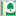Parkland College

The Fibonacci Sequence, Arik Avagyan

A with Honors Projects

A review was made of the Fibonacci sequence, its characteristics and applications.

Right Focal Boundary Value Problems For Difference Equations, 2010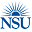Baylor University

Right Focal Boundary Value Problems For Difference Equations, Johnny Henderson, Xueyan Liu, Jeffrey W. Lyons, Jeffrey T. Neugebauer

Mathematics Faculty Articles

An application is made of a new Avery et al. fixed point theorem of compression and expansion functional type in the spirit of the original fixed point work of Leggett and Williams, to obtain positive solutions of the second order right focal discrete boundary value problem. In the application of the fixed point theorem, neither the entire lower nor entire upper boundary is required to be mapped inward or outward. A nontrivial example is also Provided.

2010Utah State University

A Comparison Of Prediction Methods Of Functional Autoregressive Time Series, Devin Didericksen

All Graduate Plan B and other Reports

Functional data analysis (FDA) is a relatively new branch of statistics that has seen a lot of expansion recently. With the advent of computer processing power and more efficient software packages we have entered the beginning stages of applying FDA methodology and techniques to data. Part of this undertaking should include an empirical assessment of the effectiveness of some of the tools of FDA, which are sound on theoretical grounds. In a small way, this project helps advance this objective.

This work begins by introducing FDA, scalar prediction techniques, and the functional autoregressive model of order one - FAR(1). Two ...

Generalized Complex Hamiltonian Torus Actions: Examples And Constraints, 2010Mathematical Institute

Generalized Complex Hamiltonian Torus Actions: Examples And Constraints, Thomas Baird, Yi Lin

Mathematical Sciences Faculty Publications

Consider an effective Hamiltonian torus action T×MM on a topologically twisted,generalized complex manifold M of dimension 2n. We prove that the rank(T)≤n−2 and that the topological twisting survives Hamiltonian reduction. We then construct a large new class of such actions satisfying rank(T)=n−2, using a surgery procedure on toric manifolds.

Fejér Polynomials And Control Of Nonlinear Discrete Systems, 2010Odessa National Polytechnic University

Fejér Polynomials And Control Of Nonlinear Discrete Systems, Dmitriy Dmitrishin, Paul Hagelstein, Anna Khamitova, Anatolii Korenovskyi, Alexander M. Stokolos

Mathematical Sciences Faculty Publications

We consider optimization problems associated to a delayed feedback control (DFC) mechanism for stabilizing cycles of one dimensional discrete time systems. In particular, we consider a delayed feedback control for stabilizing T-cycles of a differentiable function f : R → R of the form x(k + 1) = f(x(k)) + u(k) where u(k) = (a1−1)f(x(k))+a2f(x(k−T))+· · ·+aN f(x(k−(N −1)T)) , with a1 + · · · + aN = 1. Following an approach of Morgul, we associate to each periodic orbit of f, N ∈ N, and a1, . . . , aN an ...

2010Georgia Southern University

Rademacher-Type Formulas For Partitions And Overpartitions, Andrew Sills

Mathematical Sciences Faculty Publications

A Rademacher-type convergent series formula which generalizes the Hardy-Ramanujan-Rademacher formula for the number of partitions of n and the Zuckerman formula for the Fourier coefficients of ϑ4_0 | τ_−1 is presented.

Some Implications Of Chu's 10Ψ10 Generalization Of Bailey's 6Ψ6 Summation Formula, 2010West Chester University of Pennsylvania

Some Implications Of Chu's 10Ψ10 Generalization Of Bailey's 6Ψ6 Summation Formula, James Mclaughlin, Andrew Sills, Peter Zimmer

Mathematical Sciences Faculty Publications

Lucy Slater used Bailey's 6ψ6 summation formula to derive the Bailey pairs she used to construct her famous list of 130 identities of the Rogers-Ramanujan type.

In the present paper we apply the same techniques to Chu's 10ψ10 generalization of Bailey's formula to produce quite general Bailey pairs. Slater's Bailey pairs are then recovered as special limiting cases of these more general pairs.

In re-examining Slater's work, we find that her Bailey pairs are, for the most part, special cases of more general Bailey pairs containing one or more free parameters ...

Numerical Investigation Of Aeroelastic Mode Distribution For Aircraft Wing Model In Subsonic Air Flow, 2010University of New Hampshire

Numerical Investigation Of Aeroelastic Mode Distribution For Aircraft Wing Model In Subsonic Air Flow, Marianna A. Shubov, Stephen B. Wineberg, Robert Holt

Mathematics & Statistics Scholarship

In this paper, the numerical results on two problems originated in aircraft wing modeling have been presented. The first problem is concerned with the approximation to the set of the aeroelastic modes, which are the eigenvalues of a certain boundary-value problem. The affirmative answer is given to the following question: can the leading asymptotical terms in the analytical formulas be used as reasonably accurate description of the aeroelastic modes? The positive answer means that these leading terms can be used by engineers for practical calculations. The second problem is concerned with the flutter phenomena in aircraft wings in a subsonic ...

Diagonal Forms And The Rationality Of The Poincaré Series, 2010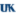University of Kentucky

Diagonal Forms And The Rationality Of The Poincaré Series, Dibyajyoti Deb

University of Kentucky Doctoral Dissertations

The Poincaré series, Py(f) of a polynomial f was first introduced by Borevich and Shafarevich in [BS66], where they conjectured, that the series is always rational. Denef and Igusa independently proved this conjecture. However it is still of interest to explicitly compute the Poincaré series in special cases. In this direction several people looked at diagonal polynomials with restrictions on the coefficients or the exponents and computed its Poincaré series. However in this dissertation we consider a general diagonal polynomial without any restrictions and explicitly compute its Poincaré series, thus extending results of Goldman, Wang and Han. In a ...

General Flips And The Cd-Index, 2010University of Kentucky

General Flips And The Cd-Index, Daniel J. Wells

University of Kentucky Doctoral Dissertations

We generalize bistellar operations (often called flips) on simplicial manifolds to a notion of general flips on PL-spheres. We provide methods for computing the cd-index of these general flips, which is the change in the cd-index of any sphere to which the flip is applied. We provide formulas and relations among flips in certain classes, paying special attention to the classic case of bistellar flips. We also consider questions of "flip-connecticity", that is, we show that any two polytopes in certain classes can be connected via a sequence of flips in an appropriate class.

Algorithms For Upper Bounds Of Low Dimensional Group Homology, 2010University of Kentucky

Algorithms For Upper Bounds Of Low Dimensional Group Homology, Joshua D. Roberts

University of Kentucky Doctoral Dissertations

A motivational problem for group homology is a conjecture of Quillen that states, as reformulated by Anton, that the second homology of the general linear group over R = Z[1/p; ζp], for p an odd prime, is isomorphic to the second homology of the group of units of R, where the homology calculations are over the field of order p. By considering the group extension spectral sequence applied to the short exact sequence 1 → SL2GL2GL1 → 1 we show that the calculation of the homology of SL2 gives information about this conjecture. We ...

Upper Bounds On The Splitting Of The Eigenvalues, 2010University of Kentucky

Upper Bounds On The Splitting Of The Eigenvalues, Phuoc L. Ho

University of Kentucky Doctoral Dissertations

We establish the upper bounds for the difference between the first two eigenvalues of the relative and absolute eigenvalue problems. Relative and absolute boundary conditions are generalization of Dirichlet and Neumann boundary conditions on functions to differential forms respectively. The domains are taken to be a family of symmetric regions in Rn consisting of two cavities joined by a straight thin tube. Our operators are Hodge Laplacian operators acting on k-forms given by the formula Δ(k) = +δd, where d and δ are the exterior derivatives and the codifferentials respectively. A result has been established on Dirichlet ...

Unitary Equivalence To A Complex Symmetric Matrix: Geometric Criteria, 2010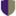Pomona College

Unitary Equivalence To A Complex Symmetric Matrix: Geometric Criteria, Levon Balayan '09, Stephan Ramon Garcia

Pomona Faculty Publications and Research

We develop several methods, based on the geometric relationship between the eigenspaces of a matrix and its adjoint, for determining whether a square matrix having distinct eigenvalues is unitarily equivalent to a complex symmetric matrix. Equivalently, we characterize those matrices having distinct eigenvalues which lie in the unitary orbit of the complex symmetric matrices.

2010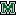Marshall University

Mathematics In Motion: Linear Systems Of Differential Equations On The Differential Analyzer, Devon A. Tivener

Theses, Dissertations and Capstones

In this work, I will provide an introduction to the dierential analyzer, a machine designed to solve dierential equations through a process called mechanical integration. I will give a brief historical account of dierential analyzers of the past, and discuss the Marshall University Dierential Analyzer Project. The goal of this work is to provide an analysis of solutions of systems of dierential equations using a dierential analyzer. In particular, we are interested in the points at which these systems are in equilibrium and the behavior of solutions that start away from equilibrium. After giving a description of linear systems of ...

Effects Of Multitemperature Nonequilibrium On Compressible Homogeneous Turbulence, 2010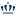Old Dominion University

Effects Of Multitemperature Nonequilibrium On Compressible Homogeneous Turbulence, Wei Liao, Yan Peng, Li-Shi Luo

Mathematics & Statistics Faculty Publications

We study the effects of the rotational-translational energy exchange on the compressible decaying homogeneous isotropic turbulence (DHIT) in three dimensions through direct numerical simulations. We use the gas-kinetic scheme coupled with multitemperature nonequilibrium based on the Jeans-Landau-Teller model. We investigate the effects of the relaxation time of rotational temperature, ZR, and the initial ratio of the rotational and translational temperatures, TR0 / TL0, on the dynamics of various turbulence statistics including the kinetic energy K (t), the dissipation rate ε (t), the energy spectrum E (k,t), the root mean square of the velocity divergence θ′ (t), the ...

Modeling Super-Spreading Events For Sars, 2010Marshall University

Modeling Super-Spreading Events For Sars, Thembinkosi P. Mkhatshwa

Theses, Dissertations and Capstones

One of the intriguing characteristics of the 2003 severe acute respiratory syndrome (SARS) epidemics was the occurrence of super spreading events (SSEs). Super-spreading events for a specific infectious disease occur when infected individuals infect more than the average number of secondary cases. The understanding of these SSEs is critical to under- standing the spread of SARS. In this thesis, we present a modification of the basic SIR (Susceptible - Infected - Removed) disease model, an SIPR (Susceptible - Regular Infected - Super-spreader - Removed) model, which captures the effect of the SSEs.